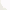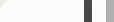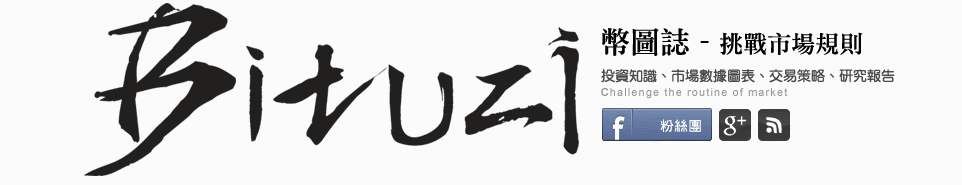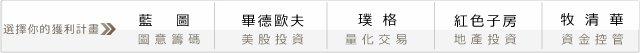## 坊間大絕開盤八法的程式化策略剖析K棒的形式有3種，黑K、紅K跟十字K棒。

3根K棒總共有2×2×2=8種組合，

(1). 漲漲漲

(2). 漲漲跌

(3). 漲跌跌

(4). 跌跌跌

(5). 跌跌漲

(6). 跌漲漲

(7). 漲跌漲

(8). 跌漲跌

(1). 漲漲漲－多空：多。
(2). 漲漲跌－多空：多。
(3). 漲跌跌－多空：空。
(4). 跌跌跌－多空：空。
(5). 跌跌漲－多空：多。
(6). 跌漲漲－多空：多。
(7). 漲跌漲－多空：╳。
(8). 跌漲跌－多空：╳。

#### 回測時間，SHOW TIME!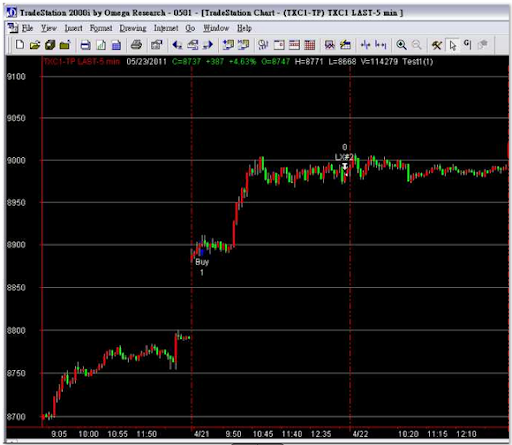input :stoploss(60);
var : buyflag(false),count(0);

if date <> date then begin
buyflag=false;
count=0;
end;

count=count+1;
if count=3 then begin
if c>o and c>o and c>o then buyflag=true;
end;
if 0900 <= Time and Time <= 1300 then begin if MarketPosition = 0 and buyflag=true then begin buy next bar at high+1 stop; end; end; if marketposition >0 then exitlong at entryprice-stoploss stop ;
if time>1339 then begin
exitlong this bar on close;
end;
setexitonclose;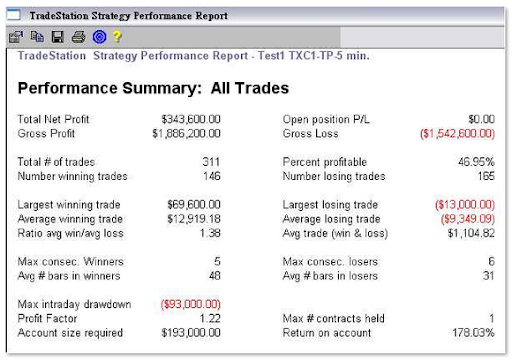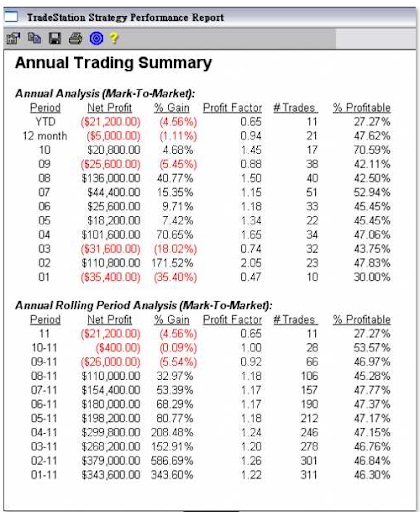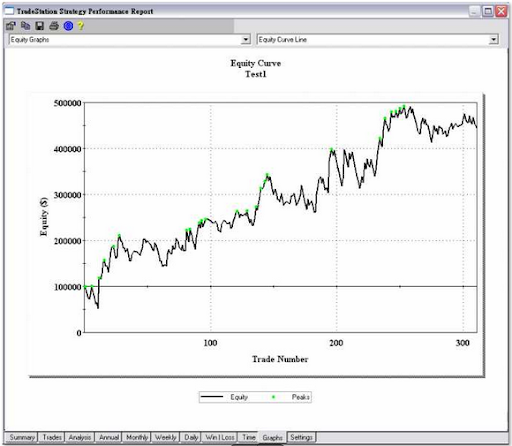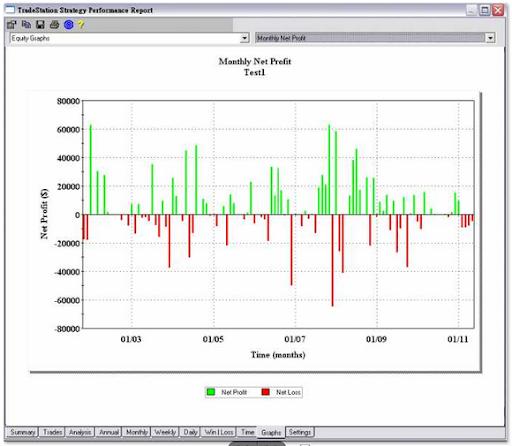#### 0 意見: